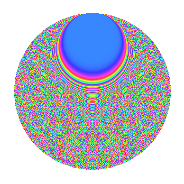# Properties

 Label 3150.2.dhLevel 3150 Weight 2 Character orbit dh Rep. character $$\chi_{3150}(479,\cdot)$$ Character field $$\Q(\zeta_{30})$$ Dimension 1920 Sturm bound 1440

# Related objects

## Defining parameters

 Level: $$N$$ = $$3150 = 2 \cdot 3^{2} \cdot 5^{2} \cdot 7$$ Weight: $$k$$ = $$2$$ Character orbit: $$[\chi]$$ = 3150.dh (of order $$30$$ and degree $$8$$) Character conductor: $$\operatorname{cond}(\chi)$$ = $$1575$$ Character field: $$\Q(\zeta_{30})$$ Sturm bound: $$1440$$

## Dimensions

The following table gives the dimensions of various subspaces of $$M_{2}(3150, [\chi])$$.

Total New Old
Modular forms 5824 1920 3904
Cusp forms 5696 1920 3776
Eisenstein series 128 0 128

## Trace form

 $$1920q - 480q^{4} + O(q^{10})$$ $$1920q - 480q^{4} + 38q^{15} - 480q^{16} - 12q^{21} + 10q^{30} + 30q^{35} - 4q^{39} - 96q^{45} + 36q^{50} - 24q^{51} - 2q^{60} + 180q^{62} + 50q^{63} - 480q^{64} - 126q^{69} + 24q^{70} + 204q^{75} - 240q^{77} - 24q^{79} - 44q^{81} + 18q^{84} - 90q^{89} - 216q^{90} + 60q^{92} + 32q^{99} + O(q^{100})$$

## Decomposition of $$S_{2}^{\mathrm{new}}(3150, [\chi])$$ into newform subspaces

The newforms in this space have not yet been added to the LMFDB.

## Decomposition of $$S_{2}^{\mathrm{old}}(3150, [\chi])$$ into lower level spaces

$$S_{2}^{\mathrm{old}}(3150, [\chi]) \cong$$ $$S_{2}^{\mathrm{new}}(1575, [\chi])$$$$^{\oplus 2}$$

## Hecke Characteristic Polynomials

There are no characteristic polynomials of Hecke operators in the database